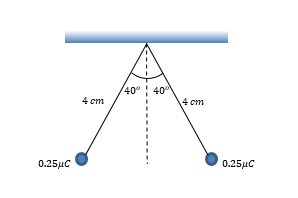# Problem: Two identical, 0.25μC charges hang from the end of electroscope leaves. If, in equilibrium, the electroscope leaves deflect from one another to an angle of 40° from the horizontal axis, as shown in the figure, what is the mass of each charge?

###### FREE Expert Solution
98% (488 ratings)
###### Problem Details

Two identical, 0.25μC charges hang from the end of electroscope leaves. If, in equilibrium, the electroscope leaves deflect from one another to an angle of 40° from the horizontal axis, as shown in the figure, what is the mass of each charge?